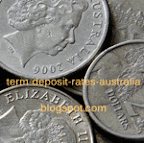## Jul 2, 2011

### BOQ TD Interest Rates

Term Deposit Rates Australia: BOQ TD Interest RatesFrom Term Deposit Rates Australia

At Term Deposit Rates Australia we share details of BOQ TD Interest Rates with Australian and non-Australian visitors.

Kindly note that BOQ TD interest rates Australia are subject to change without notice at any time at the discretion of the bank. BOQ TD Interest Rates quoted are indicative rates only.

You should verify BOQ TD Interest rates Australia with the bank concerned before using the information that we provide for any financial investment.

Here is the list of BOQ TD Interest Rates listing.

BOQ TD Interest Rates:

Term in months  | \$20,000 to  \$49,999

Less than 1  =0.70
1 less than 2  =3.20
2 less than 3  =3.20
3 less than 4  =3.20
4 less than 5  =5.80
5 less than 6  =3.40
6 less than 7  =6.05
7 less than 8  =3.40
8 less than 9  =3.50
9 less than 10  =6.15
10 less than 11  =3.60
11 less than 12  =3.70
12 less than 24  =6.25
24 less than 36  =6.35
36 less than 48  =6.50
48 less than 60  =5.00

We hope you find our Term Deposit Rates Australia useful and of interest to you.